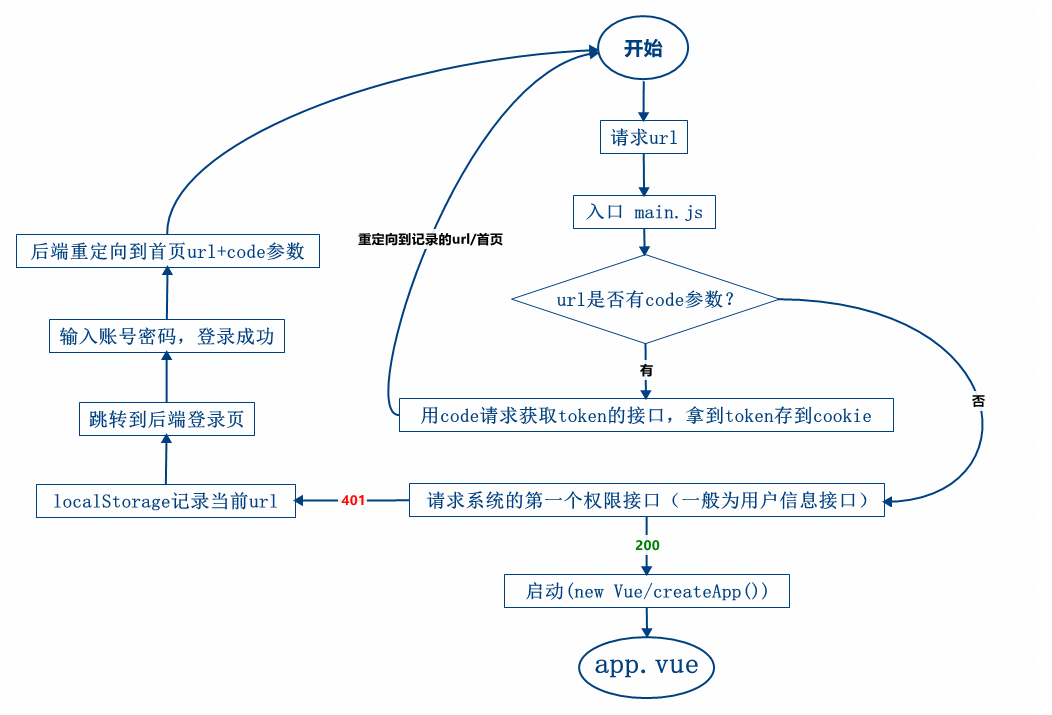# vue单点登录的实现方式sso之以code换token

## 基本实现逻辑• a、url有code，则需要将code值传给后端授权接口验证真伪，真则通过授权，登录成功；
• b、url没有code，如果请求正常接口为401，则说明未登录，跳去登录页，如果正常接口请求为200，说明已经登录成功，前端此时启动Vue；

## 简单示例（vue2.x）：

main.js

``````import Vue from 'vue'
import { start } from '@/utils/sso.js'
import App from './App'
import store from './store'
import router from './router'

function setup(){
new Vue({
el: '#app',
router,
store,
render: h => h(App)
})
}

start(()=>{
setup()
})
``````

sso.js

``````import Cookies from 'js-cookie'
import axios from 'axios'
import user from '@/api/user'

export function start(next){
let code = getQueryVariable('code')
if(code){
getTokenByCode(code, next)
}else{
user.getUserInfo().then((res)=>{
typeof next==='function'?next():''
})
}
}

function getQueryVariable(variable) {
let query = window.location.search.substring(1)
let vars = query.split('&')
for (let i = 0; i < vars.length; i++) {
let pair = vars[i].split('=')
if (pair === variable) {
return pair
}
}
return ''
}
function getTokenByCode(code, next){
axios({
url: 'get/token',
data: { code },
method: 'post'
}).then((res)=>{
typeof next==='function'?next():''
})
}
``````• 前端全栈之路（微信群）• 794324979
• 734802480（已满）

﻿